Home Data Structures Sieve of Eratosthenes

# Sieve of Eratosthenes

Sieve of Eratosthenes is an algorithm to find all prime numbers up to a given range. This concept is very important when it comes to competitive programming because it is the most efficient way to find all primes smaller than n until variable n is smaller than 10 million.

```Example
n = 7
2, 3, 5, 7

n = 15
2, 3, 5, 7, 11, 13```

#### Let us understand how it works

Since a prime number have only two divisors 1 and itself. We will use this concept to find all the prime numbers up to a given range.

1. Create a list of all consecutive integers from 2 to n(n is our range).
2. Initially let p=2, the first prime number.
3. Starting from 2p and mark all the factors of p in the range 2p to n. These factors will be 2p, 3p, 4p, 5p and so on
4. Find the next unmarked number greater than p and repeat step 2. If there is no such number stop.

#### Example

Let’s understand the working of Sieve of Eratosthenes for n = 50. Here we require to print all the prime numbers in the range  2 – 50.

List of all numbers from 2 to 50. Note 1 is neither prime nor not prime.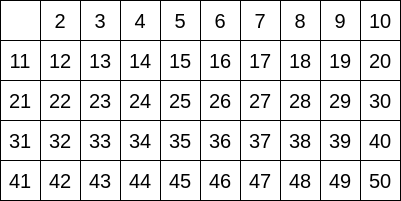Let p=2, using step 2, we will mark all the factors of 2 in range 4(2p) to 50 i.e. 2, 4, 8……50.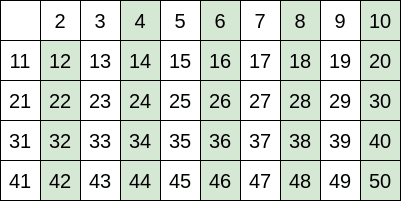Now 3 is the next, not marked integer. So, now p=3, using step 2, we will mark all the factors of 3 from 6(3*p) till 50 i.e. 6, 9…….48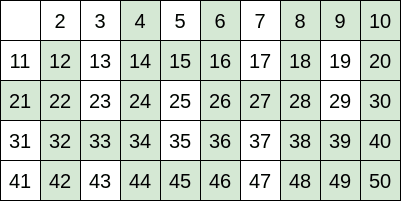Now 5 is the next, not marked integer. So, now p=5, using step 2, we will mark all the factors of 5 from 10(2*p) to 50 i.e. 10, 15 … 50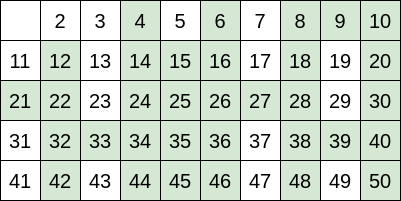We will continue this process for all the unmarked integer and our final table will look as represented below.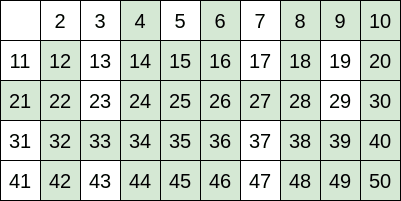So, the prime numbers in range 2 to 50 are unmarked one – 2, 3, 5, 7, 11, 13, 17, 19, 23, 29, 31, 37, 41, 43, 47.

##### Implementation of the above algorithm in CPP
```#include <bits/stdc++.h>
using namespace std;

int sieve_of_eratosthenes(int n){

vector<bool> prime(n+1,false);

for(int p=2; p<=n; p++)
{
if(prime[p]==false)
{
for(int k = 2*p; k<=n; k=k+p)
prime[k] = true;
}
}

cout<<"Prime numbers between 2 to "<<n<<":"<<endl;
for(int i=2;i<=n;i++)
if(prime[i]==false)
cout<<i<<" ";
cout<<endl;

return 0;
}

int main(){
int n = 50;
sieve_of_eratosthenes(n);

return 0;
}

```
##### Output
```Prime numbers between 2 to 50:
2 3 5 7 11 13 17 19 23 29 31 37 41 43 47```

The time complexity of the above algorithm is O(n*log(log n)).